# Ace

From complete sets of playing cards (32 cards) we pulled out one card.

What is the probability of pulling the ace?

Result

p =  12.5 %

#### Solution:Leave us a comment of example and its solution (i.e. if it is still somewhat unclear...):

Showing 0 comments:Be the first to comment!#### To solve this verbal math problem are needed these knowledge from mathematics:

Our percentage calculator will help you quickly calculate various typical tasks with percentages. Would you like to compute count of combinations?

## Next similar examples:

1. Probability of intersectionThree students have a probability of 0.7,0.5 and 0.4 to graduated from university respectively. What is the probability that at least one of them will be graduated?
2. BallsFrom the urn in which are 7 white balls and 17 red, gradually drag 3-times without replacement. What is the probability that pulls balls are in order: red red red?
3. SalesFrom statistics of sales goods, item A buy 51% of people and item B buys 59% of people. What is the probability that from 10 people buy 2 item A and 8 item B?
4. Scrap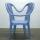From 6 products are 3 scrap. What is the probability that the random pick of 2 products have no defective product?
5. Two doctorsDoctor A will determine the correct diagnosis with a probability 86% and doctor B with a probability 86%. Calculate probability of correct diagnosis if patient is diagnosed by both doctors.
6. Lathe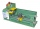95% of the components manufactured on the lathe comply with the standard, of which 80% of the components are first-class. How likely can we expect a manufactured part to be first class?
7. A jackpot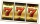How many times must I play this jackpot to win? A jackpot of seven games having (1 X 2), i. E. , home win or away win.
8. RectangleIn rectangle with sides 10 and 8 mark the diagonal. What is the probability that a randomly selected point within the rectangle is closer to the diagonal than to any side of the rectangle?
9. PediatricianPediatrician this month of 19 working days takes 3 days holidays. What is the probability that on Monday will be at work?
10. WordWhat is the probability that a random word composed of chars T, H, A, M will be MATH?
11. DicesWe will throw two dice. What is the probability that the ratio between numbers on first and second dice will be 1:2?
12. Event probability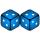The probability of event P in 8 independent experiments is 0.33. What is the probability that the event P occurs in one experiment (probability is same)?
13. Shooter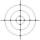The probability that a good shooter hits the center of the target circle I is 0.1. The probability that the target hit intercircle is II 0.58. What is the probability that it hits the target circle I or II?
14. Events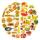Event N has probability of 0.24. What is the probability that the event N occurs in 8, 5, 4 try.
15. Pizza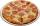A school survey found that 12 out of 15 students like pizza. If 6 students are chosen at random, what is the probability that all 6 students like pizza?
16. Bureau of Labor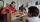Bureau of Labor is a state institution that provides mike and the rest for their so-called clients. The mission of the Bureau of Labor is spend taxpayer money to provide relaxation and benefits to those who do not want to work. Popularly speaking sense the
17. Dice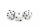How many times must throw the dice, the probability of throwing at least one six was greater than 90%?